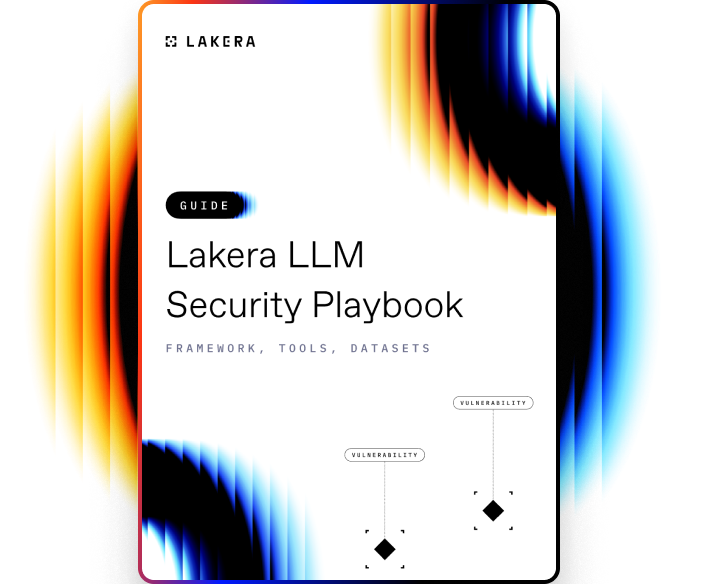VOCABULARY

# Convex Optimization

Convex optimization is a subfield of mathematical optimization that studies the problem of minimizing a convex function over a convex set. A convex function is one where the line segment between any two points on the function's graph lies above or on the graph. A convex set is a region such that, for every pair of points within the region, every point on the straight line segment that joins the pair of points is also within the region.

## How Convex Optimization works

Convex optimization works by searching within the defined convex set to locate an element that produces the smallest value of the objective function, also referred to as the optimal solution. The unique feature of convex optimization, compared to other optimization techniques, is that if there exists a local minimum that meets the provided constraints, then this solution is also the global minimum. This is due to the specific geometric properties of convex functions and sets.

The convex optimization process involves three steps:

1. Problem formulation: This involves defining the objective function to minimize, identifying the decision variables, and specifying the constraints. All these should be expressed in a convex form.
2. Problem solution: Using various algorithms like Gradient Descent, Iterative Reweighting Least Squares (IRLS), etc., the optimization problem is solved. In this phase, the algorithms iteratively refine the candidate solution to achieve an optimum.
3. Interpretation and validation of results: The final solution is checked against the problem's constraints. If it satisfies them, then the solution can be considered as the global minimum. If not, the problem formulation might need to be revised.

Convex optimization has wide applications in various fields like machine learning, statistics, data analysis, image processing, and economics due to its impressive capacity to locate global solutions within reasonable computational timeframes.Learn how to protect against the most common LLM vulnerabilities

Download this guide to delve into the most common LLM security risks and ways to mitigate them.

Related termsActivate
untouchable mode.